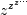# scipy.special.lambertw¶

scipy.special.lambertw(z, k=0, tol=1e-8)[source]

Lambert W function [R206].

The Lambert W function W(z) is defined as the inverse function of w * exp(w). In other words, the value of W(z) is such that z = W(z) * exp(W(z)) for any complex number z.

The Lambert W function is a multivalued function with infinitely many branches. Each branch gives a separate solution of the equation z = w exp(w). Here, the branches are indexed by the integer k.

Parameters: z : array_like Input argument. k : int, optional Branch index. tol : float, optional Evaluation tolerance. w : array w will have the same shape as z.

Notes

All branches are supported by lambertw:

• lambertw(z) gives the principal solution (branch 0)
• lambertw(z, k) gives the solution on branch k

The Lambert W function has two partially real branches: the principal branch (k = 0) is real for real z > -1/e, and the k = -1 branch is real for -1/e < z < 0. All branches except k = 0 have a logarithmic singularity at z = 0.

Possible issues

The evaluation can become inaccurate very close to the branch point at -1/e. In some corner cases, lambertw might currently fail to converge, or can end up on the wrong branch.

Algorithm

Halley’s iteration is used to invert w * exp(w), using a first-order asymptotic approximation (O(log(w)) or O(w)) as the initial estimate.

The definition, implementation and choice of branches is based on [R207].

References

 [R207] (1, 2) Corless et al, “On the Lambert W function”, Adv. Comp. Math. 5 (1996) 329-359. http://www.apmaths.uwo.ca/~djeffrey/Offprints/W-adv-cm.pdf

Examples

The Lambert W function is the inverse of w exp(w):

```>>> from scipy.special import lambertw
>>> w = lambertw(1)
>>> w
(0.56714329040978384+0j)
>>> w*exp(w)
(1.0+0j)
```

Any branch gives a valid inverse:

```>>> w = lambertw(1, k=3)
>>> w
(-2.8535817554090377+17.113535539412148j)
>>> w*np.exp(w)
(1.0000000000000002+1.609823385706477e-15j)
```

Applications to equation-solving

The Lambert W function may be used to solve various kinds of equations, such as finding the value of the infinite power tower:

```>>> def tower(z, n):
...     if n == 0:
...         return z
...     return z ** tower(z, n-1)
...
>>> tower(0.5, 100)
0.641185744504986
>>> -lambertw(-np.log(0.5)) / np.log(0.5)
(0.64118574450498589+0j)
```

#### Previous topic

scipy.special.spence

#### Next topic

scipy.special.zeta